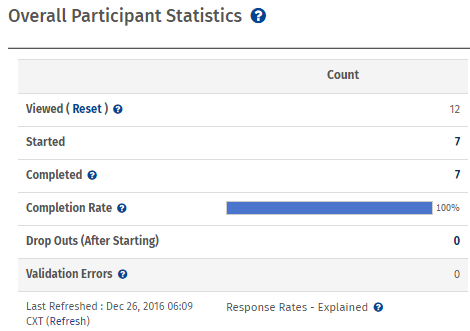Statistical terms used in reports

Various terms used in the real-time summary report or in the custom report mean

• Viewed: Number of respondents who opened the survey link.
• Started: Number of respondents who have submitted a partial response however, not completed the survey.
• Completed: Number of respondents who completed the Survey
• Completion Rate: Percentage of respondents who completed the survey after starting.
• Drop Outs (After Starting): Number or respondents who did not finish the survey after starting.
• Average Time to Complete Survey: Average time taken by respondents to complete the survey.Statistical terms.

• Mean:The mean is the arithmetic average of a set of values. Mean is calculated as the sum of all the given elements divided by the total number of elements.
• Confidence Interval: The confidence interval gives an estimated range of values which is likely to include an unknown population parameter, the estimated range being calculated for a given set of sample data collected. The width of the confidence interval gives us some idea about how uncertain we are about the unknown parameter. A very wide interval may indicate that more data should be collected before anything very definite can be said about the parameter.
• Standard Deviation: Standard deviation is a statistical measure of spread or variability. The standard deviation is the root mean square (RMS) deviation of the values from their arithmetic mean.
• Standard Error: The standard error is a method of measurement or estimation of the standard deviation of the sampling distribution associated with the estimation method.